# Orthogonal Matching Pursuit¶

Using orthogonal matching pursuit for recovering a sparse signal from a noisy measurement encoded with a dictionary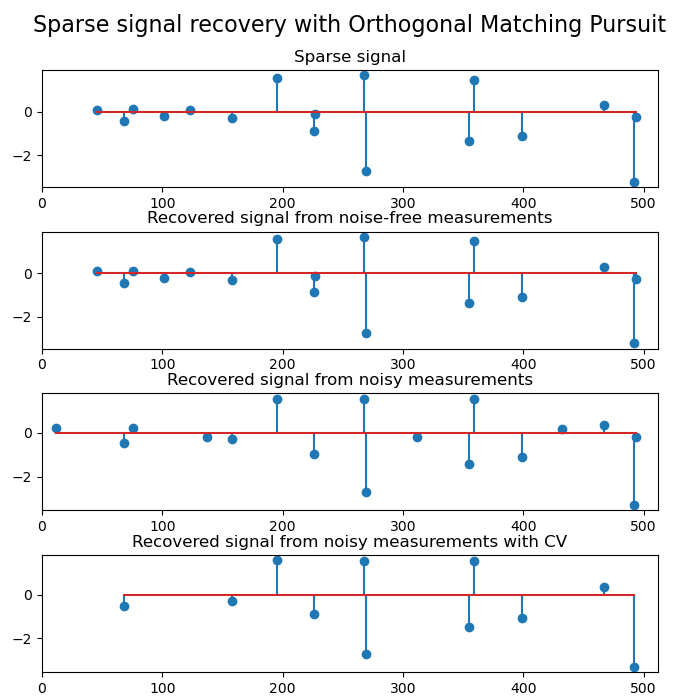import matplotlib.pyplot as plt
import numpy as np

from sklearn.datasets import make_sparse_coded_signal
from sklearn.linear_model import OrthogonalMatchingPursuit, OrthogonalMatchingPursuitCV

n_components, n_features = 512, 100
n_nonzero_coefs = 17

# generate the data

# y = Xw
# |x|_0 = n_nonzero_coefs

y, X, w = make_sparse_coded_signal(
n_samples=1,
n_components=n_components,
n_features=n_features,
n_nonzero_coefs=n_nonzero_coefs,
random_state=0,
)
X = X.T

(idx,) = w.nonzero()

# distort the clean signal
y_noisy = y + 0.05 * np.random.randn(len(y))

# plot the sparse signal
plt.figure(figsize=(7, 7))
plt.subplot(4, 1, 1)
plt.xlim(0, 512)
plt.title("Sparse signal")
plt.stem(idx, w[idx])

# plot the noise-free reconstruction
omp = OrthogonalMatchingPursuit(n_nonzero_coefs=n_nonzero_coefs)
omp.fit(X, y)
coef = omp.coef_
(idx_r,) = coef.nonzero()
plt.subplot(4, 1, 2)
plt.xlim(0, 512)
plt.title("Recovered signal from noise-free measurements")
plt.stem(idx_r, coef[idx_r])

# plot the noisy reconstruction
omp.fit(X, y_noisy)
coef = omp.coef_
(idx_r,) = coef.nonzero()
plt.subplot(4, 1, 3)
plt.xlim(0, 512)
plt.title("Recovered signal from noisy measurements")
plt.stem(idx_r, coef[idx_r])

# plot the noisy reconstruction with number of non-zeros set by CV
omp_cv = OrthogonalMatchingPursuitCV()
omp_cv.fit(X, y_noisy)
coef = omp_cv.coef_
(idx_r,) = coef.nonzero()
plt.subplot(4, 1, 4)
plt.xlim(0, 512)
plt.title("Recovered signal from noisy measurements with CV")
plt.stem(idx_r, coef[idx_r])

plt.subplots_adjust(0.06, 0.04, 0.94, 0.90, 0.20, 0.38)
plt.suptitle("Sparse signal recovery with Orthogonal Matching Pursuit", fontsize=16)
plt.show()


Total running time of the script: (0 minutes 0.216 seconds)

Related examples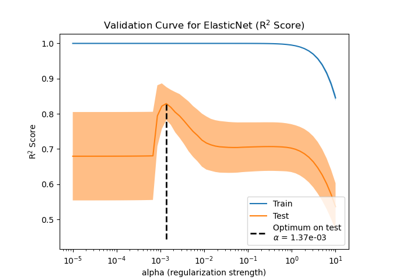Train error vs Test error

Train error vs Test error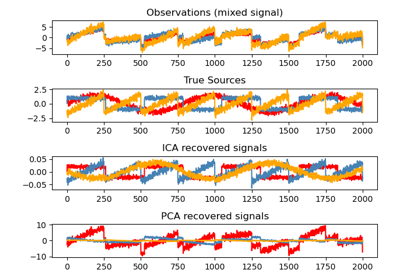Blind source separation using FastICA

Blind source separation using FastICA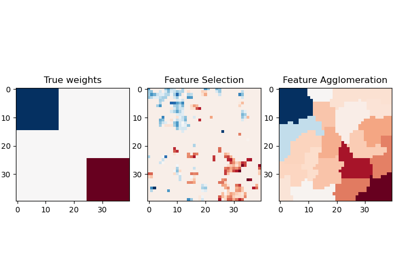Feature agglomeration vs. univariate selection

Feature agglomeration vs. univariate selection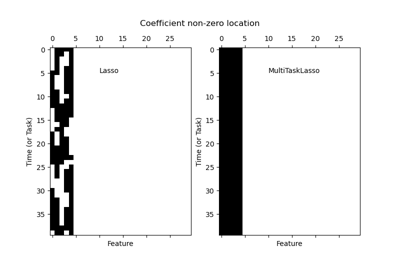Joint feature selection with multi-task Lasso

Joint feature selection with multi-task Lasso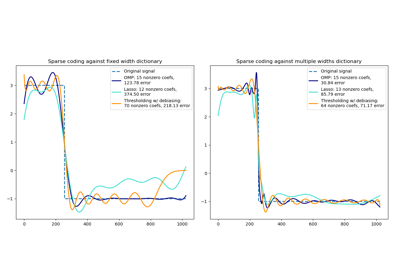Sparse coding with a precomputed dictionary

Sparse coding with a precomputed dictionary

Gallery generated by Sphinx-Gallery